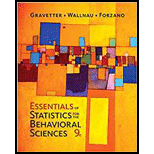Chapter 1, Problem 20PEssentials of Statistics for The B...

9th Edition
Frederick J Gravetter + 2 others
ISBN: 9781337098120

Solutions

Chapter
SectionEssentials of Statistics for The B...

9th Edition
Frederick J Gravetter + 2 others
ISBN: 9781337098120
Textbook Problem

For the following set of scores, find the value of each expression: a. ΣX b. (ΣX)2 c. ΣX2 d. Σ(X +3)a.

To determine

To Find: The value of X .

Explanation

Given info:

The values of scores X are –2, –2, 7, –3 and –1.

Calculation:

X represents first scores and X represents the sum of scores.

Software procedure:

Step-by-step procedure to obtain the X using the SPSS software:

• Go to Variable View> Enter the name of the variable as X.
• Go to Data View>Enter the values of X.
• Choose Analyze > Descriptive Statistics> choose Descriptive.
• Select X and move it under variable(s)>Choose Options>Select Sum

b.

To determine

To Find: The value of (X)2 .

c.

To determine

To Find: The value of X2 .

d.

To determine

To Find: The value of (X+3) .

Still sussing out bartleby?

Check out a sample textbook solution.

See a sample solution

The Solution to Your Study Problems

Bartleby provides explanations to thousands of textbook problems written by our experts, many with advanced degrees!

Get Started

Convert the expressions in Exercises 31-36 to positive exponent form. 10.3x265x1

Finite Mathematics and Applied Calculus (MindTap Course List)

Find y and y. y=1sect

Single Variable Calculus: Early Transcendentals, Volume I

In Exercise 9-16, find the steady state vector for the transition matrix. [0.910.10]

Finite Mathematics for the Managerial, Life, and Social Sciences

True or False: is a convergent series.

Study Guide for Stewart's Single Variable Calculus: Early Transcendentals, 8th